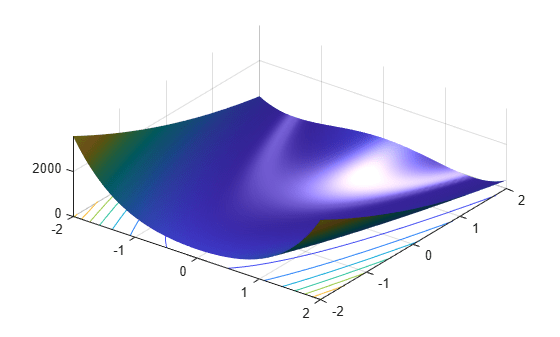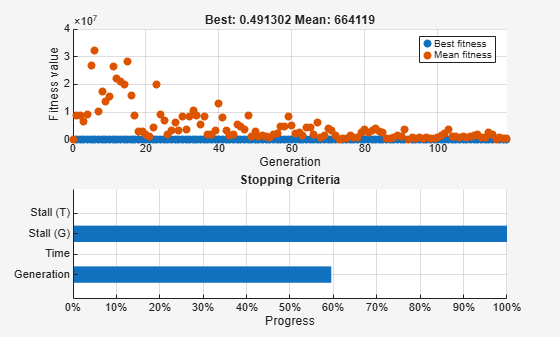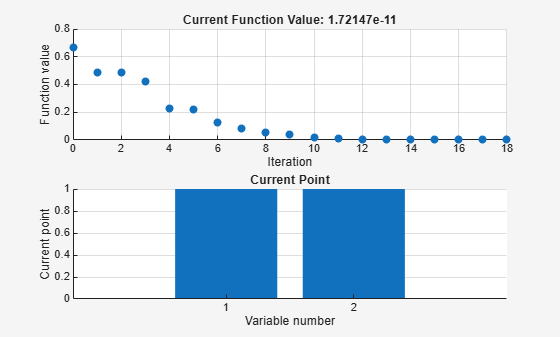Documentation

### This is machine translation

Mouseover text to see original. Click the button below to return to the English version of the page.

## Hybrid Scheme in the Genetic Algorithm

### Introduction

This example shows how to use a hybrid scheme to optimize a function using the Genetic Algorithm and another optimization method. `ga` can reach the region near an optimum point relatively quickly, but it can take many function evaluations to achieve convergence. A commonly used technique is to run `ga` for a small number of generations to get near an optimum point. Then the solution from `ga` is used as an initial point for another optimization solver that is faster and more efficient for local search.

### Rosenbrock's Function

In this example we will optimize Rosenbrock's function (also known as Dejong's second function):

``` f(x)= 100*(x(2)-x(1)^2)^2+(1-x(1))^2 ```

This function is notorious in optimization because of the slow convergence most methods exhibit when trying to minimize this function. This function has a unique minimum at the point x* = (1,1) where it has a function value f(x*) = 0.

We can view the code for this fitness function.

`type dejong2fcn.m`
```function scores = dejong2fcn(pop) %DEJONG2FCN Compute DeJongs second function. %This function is also known as Rosenbrock's function % Copyright 2003-2004 The MathWorks, Inc. scores = zeros(size(pop,1),1); for i = 1:size(pop,1) p = pop(i,:); scores(i) = 100 * (p(1)^2 - p(2)) ^2 + (1 - p(1))^2; end ```

We use the function `plotobjective` in the toolbox to plot the function `dejong2fcn` over the range = [-2 2;-2 2].

`plotobjective(@dejong2fcn,[-2 2;-2 2]);`### Genetic Algorithm Solution

To start, we will use the Genetic Algorithm, `ga`, alone to find the minimum of Rosenbrock's function. We need to supply `ga` with a function handle to the fitness function `dejong2fcn.m`. Also, `ga` needs to know the how many variables are in the problem, which is two for this function.

```FitnessFcn = @dejong2fcn; numberOfVariables = 2;```

Some plot functions can be selected to monitor the performance of the solver.

`options = optimoptions(@ga,'PlotFcn',{@gaplotbestf,@gaplotstopping});`

We set the random number stream for reproducibility, and run `ga` with the above inputs.

```rng('default') [x,fval] = ga(FitnessFcn,numberOfVariables,[],[],[],[],[],[],[],options)``````Optimization terminated: average change in the fitness value less than options.FunctionTolerance. ```
```x = 1×2 0.3454 0.1444 ```
```fval = 0.4913 ```

The global optimum is at x* = (1,1). `ga` found a point near the optimum, but could not get a more accurate answer with the default stopping criteria. By changing the stopping criteria, we might find a more accurate solution, but it may take many more function evaluations to reach x* = (1,1). Instead, we can use a more efficient local search that starts where `ga` left off. The hybrid function field in `ga` provides this feature automatically.

We will use a hybrid function to solve the optimization problem, i.e., when `ga` stops (or you ask it to stop) this hybrid function will start from the final point returned by `ga`. Our choices are `fminsearch`, `patternsearch`, or `fminunc`. Since this optimization example is smooth, i.e., continuously differentiable, we can use the `fminunc` function from Optimization Toolbox as our hybrid function. Since `fminunc` has its own options structure, we provide it as an additional argument when specifying the hybrid function.

```fminuncOptions = optimoptions(@fminunc,'Display','iter','Algorithm','quasi-newton'); options = optimoptions(options,'HybridFcn',{@fminunc, fminuncOptions});```

Run `ga` solver again with `fminunc` as the hybrid function.

`[x,fval] = ga(FitnessFcn,numberOfVariables,[],[],[],[],[],[],[],options)````Optimization terminated: average change in the fitness value less than options.FunctionTolerance. First-order Iteration Func-count f(x) Step-size optimality 0 3 0.664192 28.1 1 12 0.489131 0.000402247 0.373 2 21 0.48383 91 1.22 3 24 0.422036 1 6.7 4 33 0.225633 0.295475 7.36 5 39 0.221682 0.269766 10.1 6 45 0.126376 10 7.96 7 48 0.0839643 1 0.457 8 54 0.0519836 0.5 4.56 9 57 0.0387946 1 5.37 10 60 0.0149721 1 0.85 11 63 0.00959914 1 3.45 12 66 0.0039939 1 0.662 13 69 0.00129755 1 0.348 14 72 0.000288982 1 0.634 15 75 2.29621e-05 1 0.0269 16 78 3.34554e-07 1 0.0139 17 81 5.38696e-10 1 0.000801 18 84 1.72147e-11 1 7.21e-06 Local minimum found. Optimization completed because the size of the gradient is less than the value of the optimality tolerance. ```
```x = 1×2 1.0000 1.0000 ```
```fval = 1.7215e-11 ```

The first plot shows the best and mean values of the population in every generation. The best value found by `ga` when it terminated is also shown in the plot title. When `ga` terminated, `fminunc` (the hybrid function) was automatically called with the best point found by `ga` so far. The solution `x` and `fval` is the result of using `ga` and `fminunc` together. As shown here, using the hybrid function can improve the accuracy of the solution efficiently.

﻿

Watch now